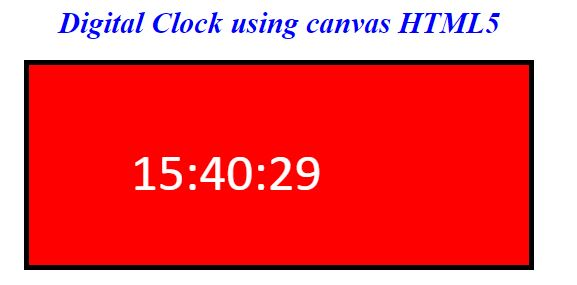# Digital clock Animation in HTML5

### Digital clock

We can create the digital clock by using the canvas HTML5.

``` <div>
<h1 style="color:blue"><i>Digital Clock using canvas HTML5</i></h1>
<canvas id="clock" width="500" height="200"></canvas>
</div>
<script>
var context;
var d;
var str;
function getClock()
{

d = new Date();
str = Calculate(d.getHours(), d.getMinutes(), d.getSeconds());

context = clock.getContext("2d");
context.clearRect(0, 20, 500, 200);
context.font = "40pt calibri";
context.fillStyle = "white";
context.fillText(str, 102, 125);
}

function Calculate(hour, min, sec)
{
var curTime;
if(hour < 10)
curTime = "0"+hour.toString();
else
curTime = hour.toString();

if(min < 10)
curTime += ":0"+min.toString();
else
curTime += ":"+min.toString();

if(sec < 10)
curTime += ":0"+sec.toString();
else
curTime += ":"+sec.toString();
return curTime;
}

setInterval(getClock, 1000);
</script>
```
• In the above code snippet we have defined the `digital clock` using canvas HTML5, we have taken the style sheet to give the style to the  clock to the background color of the clock as well as the border of the clock.

• We have canvas id as` clock` and the width as` 500` and height as `200`, the var as `d`, `context`, `str `defines the clock function.

• In the next line we are having the function `getclock()` to define the `date`, `hours`, `minutes`,` seconds`
• In the next line we are giving the values of the `rectangle`,` size` ,`font`, `color`, `filltext` to fill the text.

• `context.fillText(str, 102, 125);` - It defines the text as `str` is function to define the hours, minutes, seconds  with the x, y values in the text to be aligned in the rectangle.

• In the nextline we are having the function `calculate()` to get the` hours`, `minutes`, `seconds`
• In the function we are declaring the var current time to define the present timeby using conditional statements

• If the `(hour<10)`` curTime = "0"+hour.toString();` - current time is equal to the 0+hour .tostring(), else it is equal to the current hour .tostring()

• It the `(minute<10) cur time+ =":0"+minute.tostring();` - current time is equal to the "0"+minute.tostring(), else curTime += ":"+min.toString(); - it is equal to the min. tostring()

• If the` (second<10) curTime += ":0"+sec.toString();` - It defines the current as zero to the second.tostring(),else curTime += ":"+sec.toString(); - It is equal to the second to.string value

• The return `curtime` defines the current time

• `setInterval(getClock, 1000);` - It defines the function of the clock to the current time

outputViews: 19020 | Post Order: 118